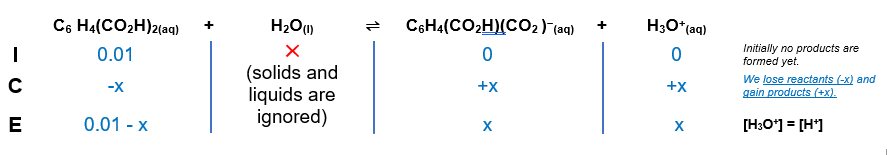# Problem: Calculate the concentration of each species present in a 0.010-M solution of phthalic acid, C6H4(CO2H)2.C6H4(CO2 H)2(aq) + H2 O(l) ⇌ H3 O+(aq) + C6 H4 (CO2 H) (CO2 )−(aq)        Ka = 1.1 × 10−3C6H4(CO2 H)(CO2)(aq) + H2 O(l) ⇌ H3O+(aq) + C6 H4(CO2)2 2−(aq)            Ka = 3.9 × 10−6

###### FREE Expert Solution

We are being asked to calculate each species present in a 0.010-M solution of phthalic acid, C6H4(CO2H)2.

Step 1: Construct an ICE chart for the equilibrium reaction.

Since we’re dealing with a weak acid and Ka is an equilibrium expression, we will have to create an ICE chart to determine the equilibrium concentration of each species

We will use the first equilibrium reaction given:

C6 H4(CO2 H)2(aq) + H2 O(l) ⇌ H3 O+(aq) + C6 H(CO2 H) (CO)(aq)

phthalic acid (aq) + H2 O(l) ⇌ H3 O+(aq) + biphthalate (aq)Step 2: Write the Ka is an equilibrium expression.

Solids and liquids are not included in the expression

Step 3: Calculate for the equilibrium concentration.

$\mathbf{1}\mathbf{.}\mathbf{1}\mathbf{×}{\mathbf{10}}^{\mathbf{-}\mathbf{3}}\mathbf{=}\frac{\left[\mathbf{x}\right]\left[\mathbf{x}\right]}{\left[\mathbf{0}\mathbf{.}\mathbf{01}\mathbf{-}\mathbf{x}\right]}\phantom{\rule{0ex}{0ex}}\mathbf{1}\mathbf{.}\mathbf{1}\mathbf{×}{\mathbf{10}}^{\mathbf{-}\mathbf{3}}\mathbf{=}\frac{{x}^{2}}{\left[0.01-x\right]}$

we cannot ignore x in the denominator

$\mathbf{\left(}\mathbf{1}\mathbf{.}\mathbf{1}\mathbf{x}{\mathbf{10}}^{\mathbf{-}\mathbf{3}}\mathbf{\right)}\mathbf{=}\frac{{\mathbf{x}}^{\mathbf{2}}}{\mathbf{0}\mathbf{.}\mathbf{015}\mathbf{-}\mathbf{x}}\phantom{\rule{0ex}{0ex}}\mathbf{\left(}\mathbf{1}\mathbf{.}\mathbf{1}\mathbf{x}{\mathbf{10}}^{\mathbf{-}\mathbf{3}}\mathbf{\right)}\left(\mathbf{0}\mathbf{.}\mathbf{01}\mathbf{-}\mathbf{x}\right)\mathbf{=}{\mathbf{x}}^{\mathbf{2}}\phantom{\rule{0ex}{0ex}}\overline{){{\mathbf{x}}}^{{\mathbf{2}}}{\mathbf{+}}{\mathbf{0}}{\mathbf{.}}{\mathbf{0011}}{\mathbf{x}}{\mathbf{-}}{\mathbf{0}}{\mathbf{.}}{\mathbf{000011}}{\mathbf{=}}{\mathbf{0}}}$

Now, we will use the quadratic formula to solve for x. The quadratic formula is:

$\overline{){\mathbf{x}}{\mathbf{=}}\frac{\mathbf{-}\mathbf{b}\mathbf{±}\sqrt{{\mathbf{b}}^{\mathbf{2}}\mathbf{-}\mathbf{4}\mathbf{a}\mathbf{c}}}{\mathbf{2}\mathbf{a}}}$

From the equation, we have a = 1, b = 0.011, and c = -0.000011

Solve for x using the solver function from the calculator:

x = -0.0039  (can't be negative) ; x =0.002812

Hence x = 0.002812 M = [bipthalate] = [C6 H(CO2 H) (CO)] = [H3O+]

phtalic acid = 0.010-0.002812 = 0.007188 M

Next is the 2nd equilibrium reaction given:

C6H4(CO2 H)(CO2)(aq) + H2 O(l) ⇌ H3O+(aq) + C6 H4(CO2)2−(aq)            Ka = 3.9 × 10−6

biphthalate (aq) + H2 O(l) ⇌ H3 O+(aq) + phthalate (aq)

98% (267 ratings)###### Problem Details

Calculate the concentration of each species present in a 0.010-M solution of phthalic acid, C6H4(CO2H)2.

C6H4(CO2 H)2(aq) + H2 O(l) ⇌ H3 O+(aq) + C6 H(CO2 H) (CO)(aq)        Ka = 1.1 × 10−3
C6H4(CO2 H)(CO2)(aq) + H2 O(l) ⇌ H3O+(aq) + C6 H4(CO2)2−(aq)            Ka = 3.9 × 10−6

Frequently Asked Questions

What scientific concept do you need to know in order to solve this problem?

Our tutors have indicated that to solve this problem you will need to apply the Diprotic Acid concept. You can view video lessons to learn Diprotic Acid. Or if you need more Diprotic Acid practice, you can also practice Diprotic Acid practice problems.

What professor is this problem relevant for?

Based on our data, we think this problem is relevant for Professor Kirschner's class at IU.

What textbook is this problem found in?

Our data indicates that this problem or a close variation was asked in Chemistry - OpenStax 2015th Edition. You can also practice Chemistry - OpenStax 2015th Edition practice problems.